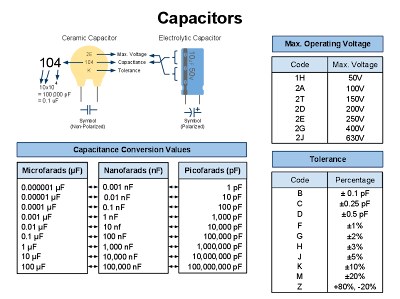## Electronic & Electrical Cheat Sheets

24 Jul 2010

Here are the first versions of the cheatsheets I've been making. These are meant to be used as quick reference guides for any electronic/electrical project.
The cheat sheets I've come up with are the following:
1. Basic Operational Amplifier Configurations
2. Capacitors
3. Ohm's & Watt's Laws
Here's a preview of the first one:The Basic Operational Amplifier Configurations cheat sheet contains the schematic diagram and transfer function for each of the following configurations:
1. Voltage Comparator
2. Non-Inverting Amplifier
3. Inverting Amplifier
4. Voltage Follower
5. Inverting Summing Amplifier
6. Differential Amplifier
7. Differentiator Amplifier
8. Integrator Amplifier

Here's a preview for the the Capacitor cheat sheet:This cheat sheet shows how to read the tolerance, maximum operating voltage and capacitance in ceramic capacitors, provides a conversion table for capacitance. Just look up the codes for the tolerance and max. voltage in the corresponding table.
In addition, I've included a drawing of an electrolytic capacitor for comparison. They are certainly not drawn to scale.

Get it here.

The third cheat sheet that I've been working on is widely available on the Internet, but since I wanted to have my own personal version, here it is: Ohm's & Watt's Laws.
They help you figure out quick and easy how to calculate current, voltage, power and resistance given two known electrical values.To view this cheat sheet, go here.

I'm going to explain to you how to use this cheat sheet in case you've never seen this before.
Let's say for example that you want to calculate how much power (in watts) will dissipate a 470 ohms resistor connected to a 9 volt battery.
The resistor is 470 ohms. Therefore R=470\ ohms.
The battery is 9 volts. So V=9\ volts.
Looking at the Watts (P) quarter in the circle (top left one), you can see that there's a formula to obtain P given V and R, which happens to be \frac{V^2}{R}.
Therefore P=\frac{V^2}{R}.
Substituting V and R in the previous formula: P=\frac{9^2}{470}=\frac{81}{470}=0.17\ watts.